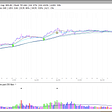# Technical Indicators Basics

## By EOD Historical Data on The Capital

We at EOD Historical Data adjust historical prices for splits and dividends (more on adjusted closes you can read on this documentation page: eodhistoricaldata.com/knowledgebase/adjusted-close-and-close-whats-the-difference/). If for some reason one only needs data adjusted for splits, but not for dividends, then this API can be used to obtain splits-only adjusted data.

## Average Volume

The average volume of a security over a period of time is the total amount traded in that period in stocks, divided by the length of the period. It is used as one of the indicators that help evaluate the performance of security on the market over a prolonged period of time and also investors’ activity around the particular asset.

E.g. AV = ( v1 + v2 + v3 ) / 3

## Simple Moving Average (SMA)

The SMA is a simple (hence its name) indicator calculated over the desired period of time on a regular basis, to monitor the performance of a security. It’s calculated easily by adding up n indicators of a stock (say, EOD close price) and dividing the sum by n. Since the name of the indicator is “moving average”, that means that it doesn’t work as a standalone indicator, but rather as a set of averages calculated on a regular basis over a period of time on corresponding data sets. That means that this indicator will change depending on dates chosen.

E. g. a stock closes at 4, 5, 5, 4, 3 during a given week. The SMA of three days over the course of these five will look like this.

SMA = ( p1 + p2 + p3 ) / 3

## Weighted Moving Average (WMA)

The WMA is an improvement over the SMA where it applies weights to different data points in the following manner: more recent data points weigh more than less recent.

E. g. WMA = ((p1 * 3) + (p2 * 2) + (p3 * 1))/ (3 + 2 + 1). Where: p1 = current price, p2 = price one bar ago, p3 = price two bars ago.

In the example from above, it will work like this.

This indicator follows the price more closely than the SMA, and it helps determine the direction of a trend; it normally works as an indication to buy when prices go downwards near or just below the WMA, or an indication to sell when prices go upwards towards or just above the WMA.

## Exponential Moving Average (EMA)

The EMA is an improvement over the WMA where it applies exponentially decreasing weights (they never get to zero) to the previous formula. It is one of the indicators used by traders to determine the direction of a trend.

This indicator follows the price more closely than the SMA, and it helps determine the direction of a trend; it normally works as an indication to buy when prices go downwards near or just below the WMA, or an indication to sell when prices go upwards towards or just above the WMA.

E. g. EMA = ( K x (C — P) ) + P

Where: C = Current Price, P = Previous period’s EMA (A SMA is used for the first period’s calculations), K = Exponential smoothing constant.

## Relative Strength Index (RSI)

The RSI is a momentum oscillator, measuring the velocity and magnitude of directional price movements. Momentum is the rate of the rise or fall in price. The RSI is typically used on a 14-day period, and measured on a scale from 0 to 100. It’s calculated as the difference between two close prices, which results in either an upward change or a downward change. A moving average is then applied to the range of both, and the SMA of upward changes is divided by SMA of downward changes; this is called “relative strength factor”. After that, all that’s left to calculate the index is 100 minus (100 divided by 1 + RSF).

Traditional usage of the RSI is as follows. Values of 70 or above indicate that a security is becoming overbought or overvalued and may be going for a trend reversal or corrective pullback in price. Values of 30 or below indicate that a security is oversold or undervalued.

--

--

--

## More from The Capital

A publishing platform for professionals in business, finance, and tech

## Two-thirds of Europeans think cryptocurrency is here for the long haul, according to a survey.## Rally Turns 1: A Look Back and Ahead## BlockFi lost over \$221 million during the 2021 Bull Run Sources | Curated CoinCodeCap #21 June 2022## Best cryptocurrency under a dollar## DeRace NFTs to be Featured on the Mintable Platform!## ONI Launch & the ONINO Farm## EODHD APIs

eodhistoricaldata.com — stock market fundamental and historical prices API for stocks, ETFs, mutual funds and bonds all over the world.

## Can You Earn Money with Seasonal Trading?## Chart Patterns for Trading Part 1## A Quick Overview of a Stock Screener to Know About## How To Trade Stocks: A Comprehensive Guide